# RS Aggarwal Solutions for Class 8 Maths Chapter 17 - Construction of Quadrilaterals Exercise 17A

Download RS Aggarwal Solutions for Class 8 Maths Chapter 17- Exercise 17A, Construction of Quadrilaterals from the links provided below. BYJU’s expert team has solved the RS Aggarwal Solutions to ensure that the students are perfect with their basic concepts by practicing the solutions. In this Exercise 17A of RS Aggarwal Class 8 Maths, we shall mainly learn how to construct different Quadrilaterals with given requirements.

## Download PDF of RS Aggarwal Solutions for Class 8 Maths Chapter 17- Construction of Quadrilaterals Exercise 17A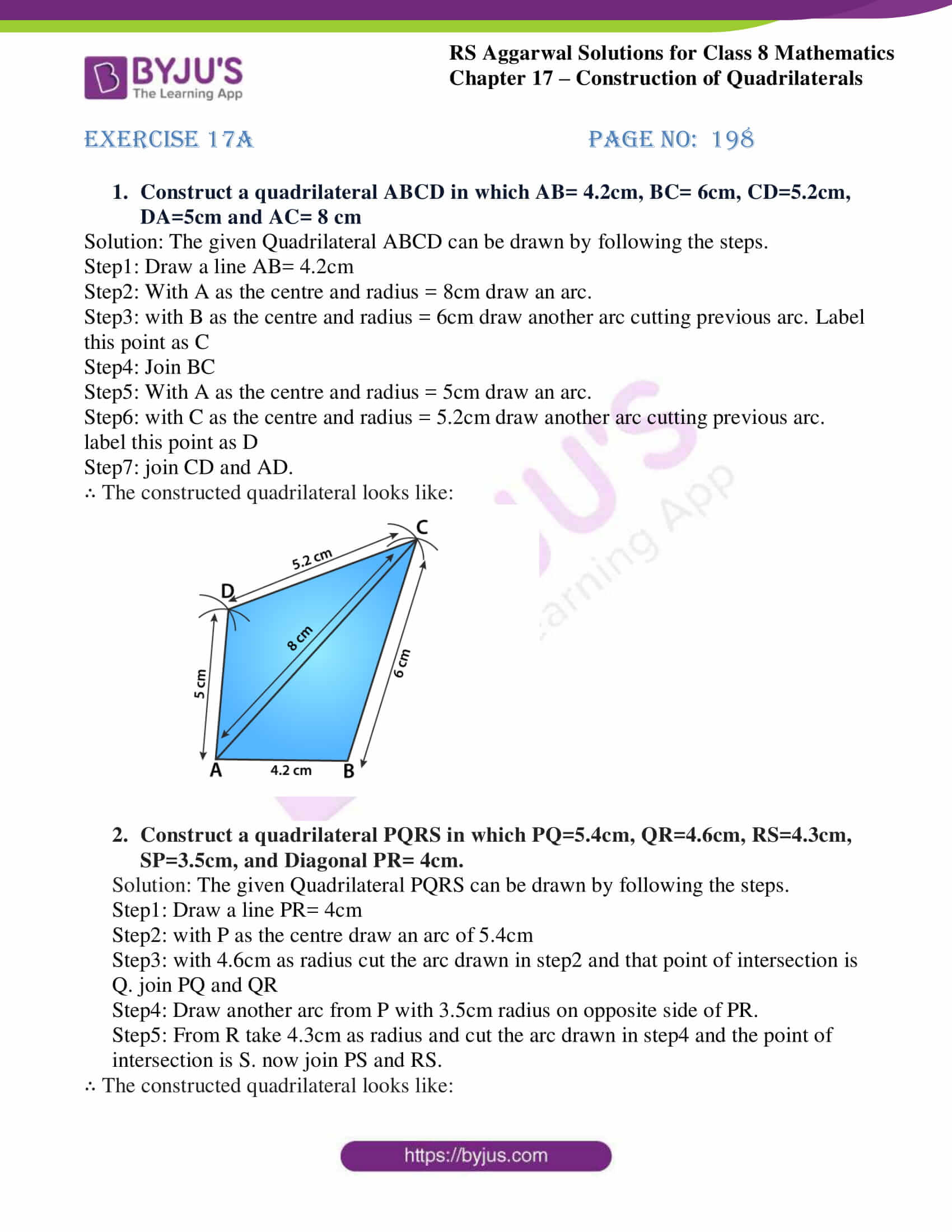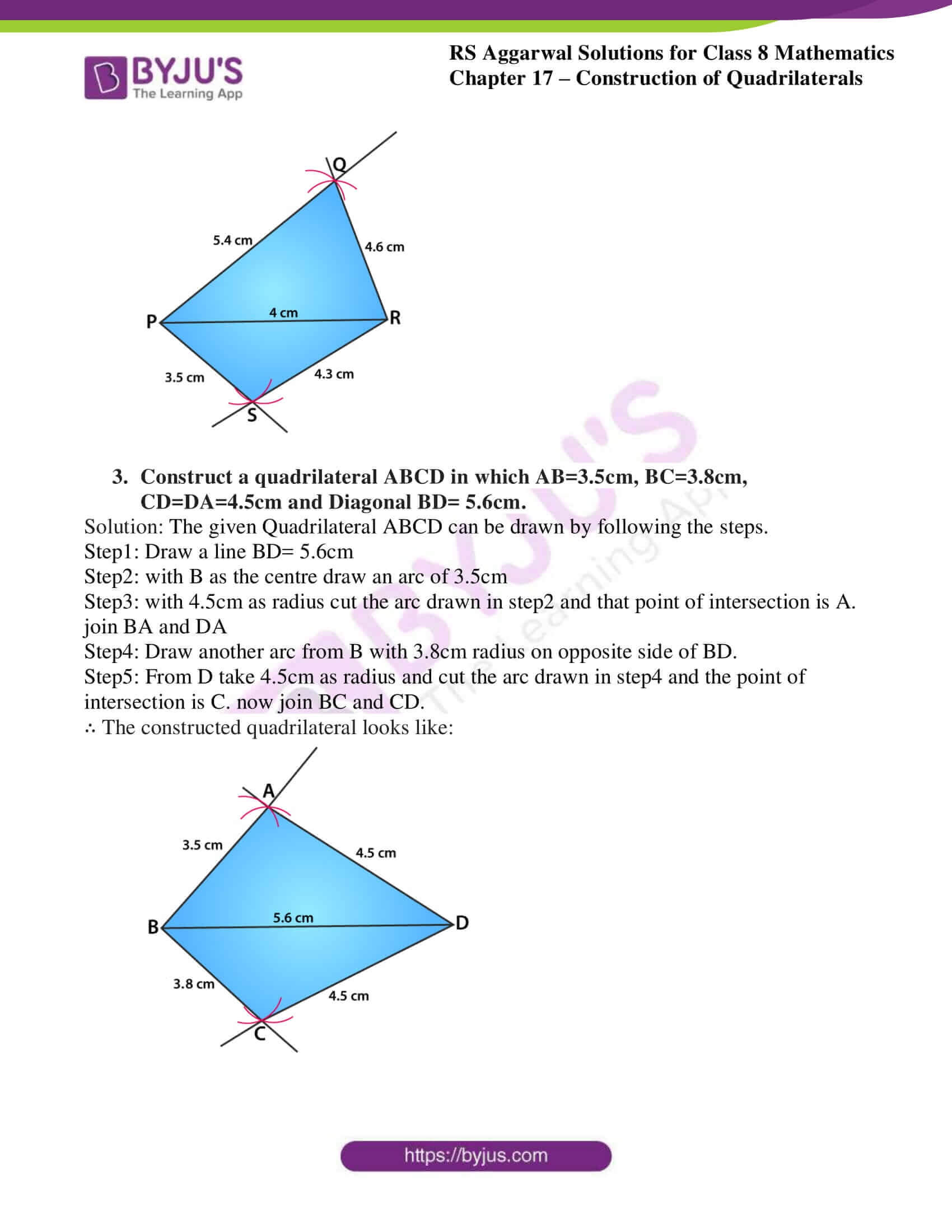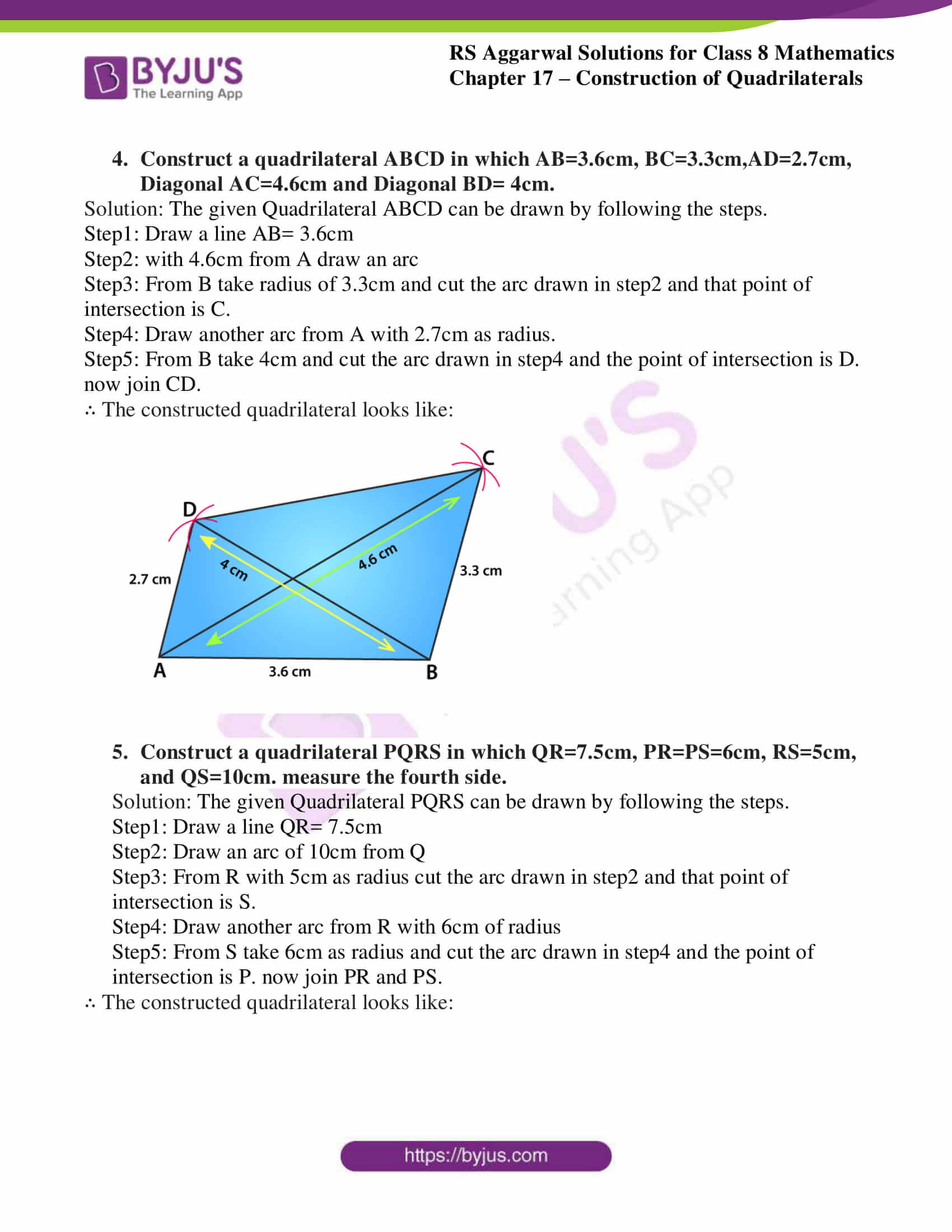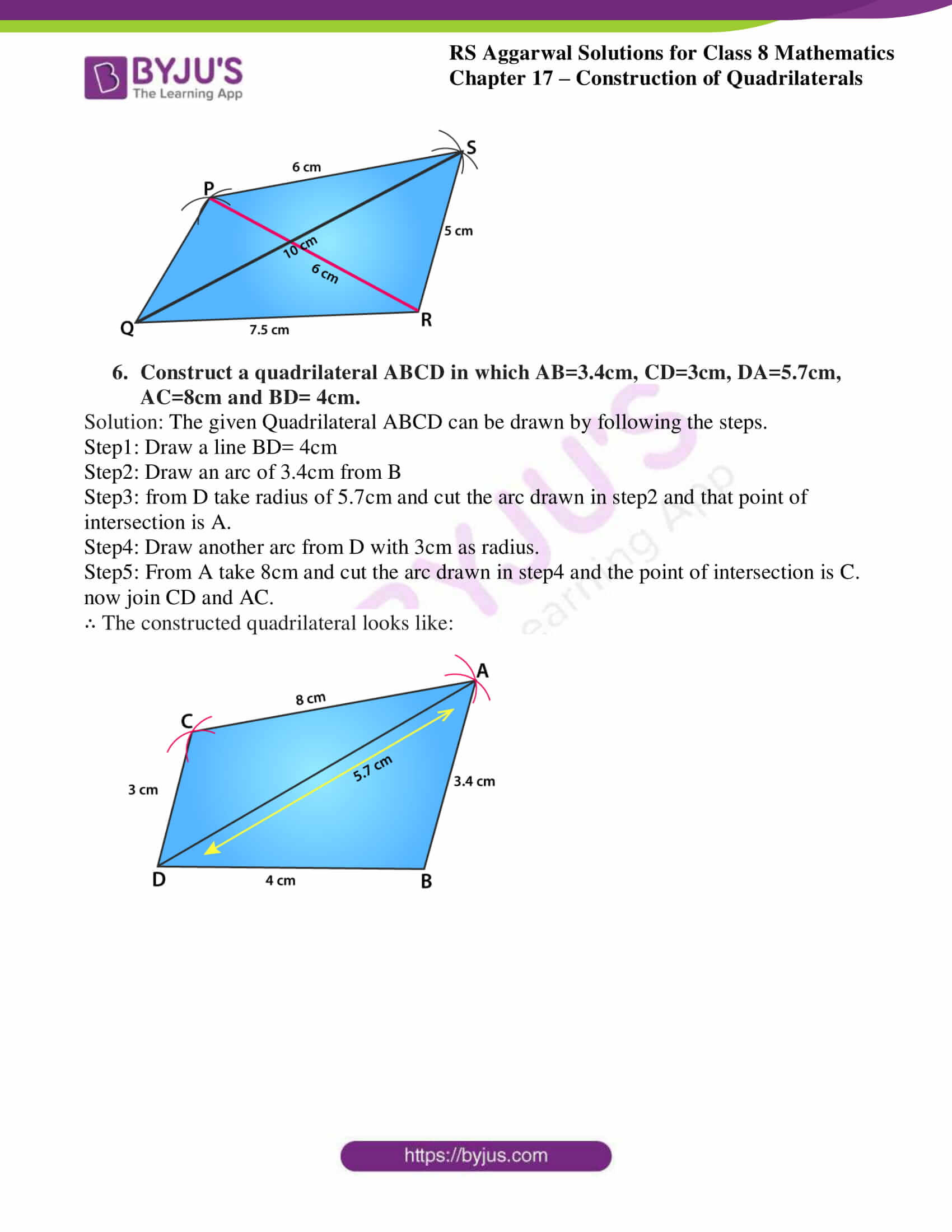### Access Answers to RS Aggarwal Solutions for Class 8 Maths Chapter 17- Construction of Quadrilaterals Exercise 17A

1. Construct a quadrilateral ABCD in which AB= 4.2cm, BC= 6cm, CD=5.2cm, DA=5cm and AC= 8 cm

Solution: The given Quadrilateral ABCD can be drawn by following the steps.

Step1: Draw a line AB= 4.2cm

Step2: With A as the centre and radius = 8cm draw an arc.

Step3: with B as the centre and radius = 6cm draw another arc cutting previous arc. Label this point as C

Step4: Join BC

Step5: With A as the centre and radius = 5cm draw an arc.

Step6: with C as the centre and radius = 5.2cm draw another arc cutting previous arc. label this point as D

∴ The constructed quadrilateral looks like: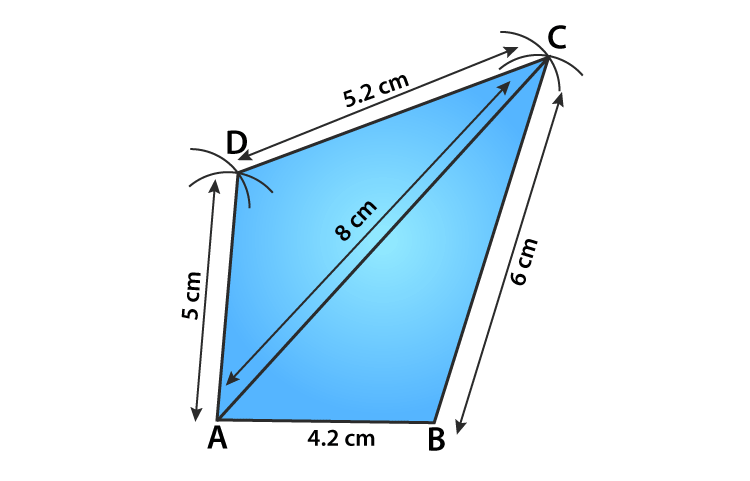2. Construct a quadrilateral PQRS in which PQ=5.4cm, QR=4.6cm, RS=4.3cm, SP=3.5cm, and Diagonal PR= 4cm.

Solution: The given Quadrilateral PQRS can be drawn by following the steps.

Step1: Draw a line PR= 4cm

Step2: with P as the centre draw an arc of 5.4cm

Step3: with 4.6cm as radius cut the arc drawn in step2 and that point of intersection is Q. join PQ and QR

Step4: Draw another arc from P with 3.5cm radius on opposite side of PR.

Step5: From R take 4.3cm as radius and cut the arc drawn in step4 and the point of intersection is S. now join PS and RS.

∴ The constructed quadrilateral looks like: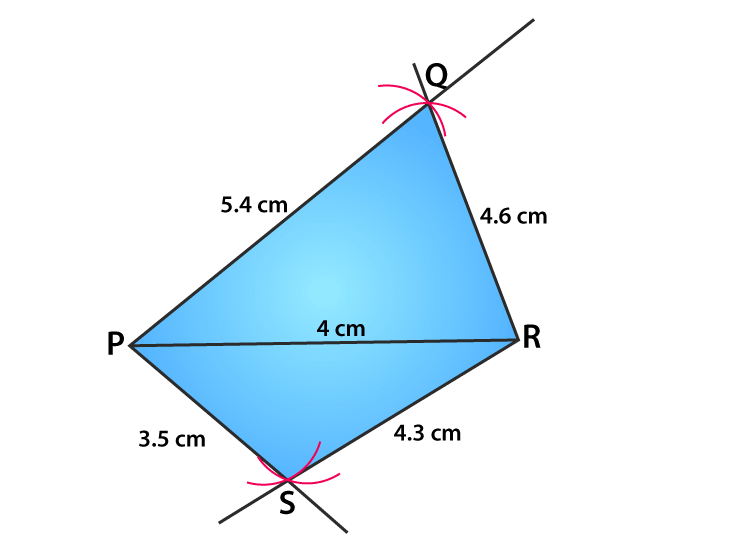3. Construct a quadrilateral ABCD in which AB=3.5cm, BC=3.8cm, CD=DA=4.5cm and Diagonal BD= 5.6cm.

Solution: The given Quadrilateral ABCD can be drawn by following the steps.

Step1: Draw a line BD= 5.6cm

Step2: with B as the centre draw an arc of 3.5cm

Step3: with 4.5cm as radius cut the arc drawn in step2 and that point of intersection is A. join BA and DA

Step4: Draw another arc from B with 3.8cm radius on opposite side of BD.

Step5: From D take 4.5cm as radius and cut the arc drawn in step4 and the point of intersection is C. now join BC and CD.

∴ The constructed quadrilateral looks like: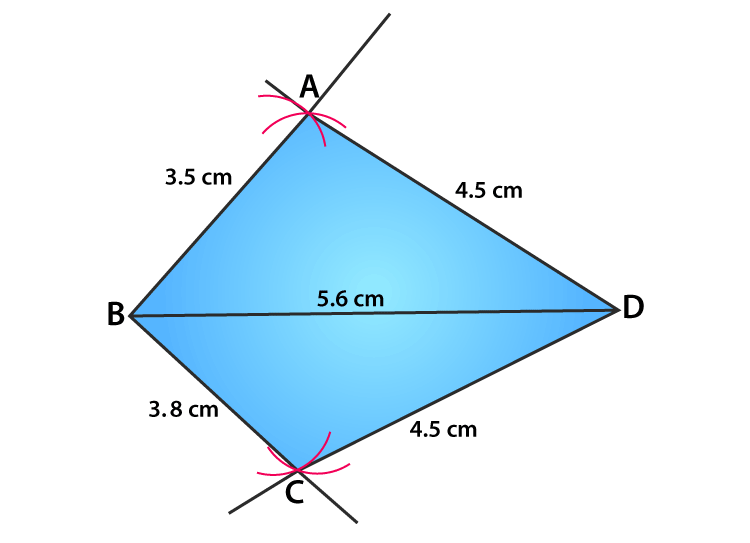4. Construct a quadrilateral ABCD in which AB=3.6cm, BC=3.3cm,AD=2.7cm, Diagonal AC=4.6cm and Diagonal BD= 4cm.

Solution: The given Quadrilateral ABCD can be drawn by following the steps.

Step1: Draw a line AB= 3.6cm

Step2: with 4.6cm from A draw an arc

Step3: From B take radius of 3.3cm and cut the arc drawn in step2 and that point of intersection is C.

Step4: Draw another arc from A with 2.7cm as radius.

Step5: From B take 4cm and cut the arc drawn in step4 and the point of intersection is D. now join CD.

∴ The constructed quadrilateral looks like: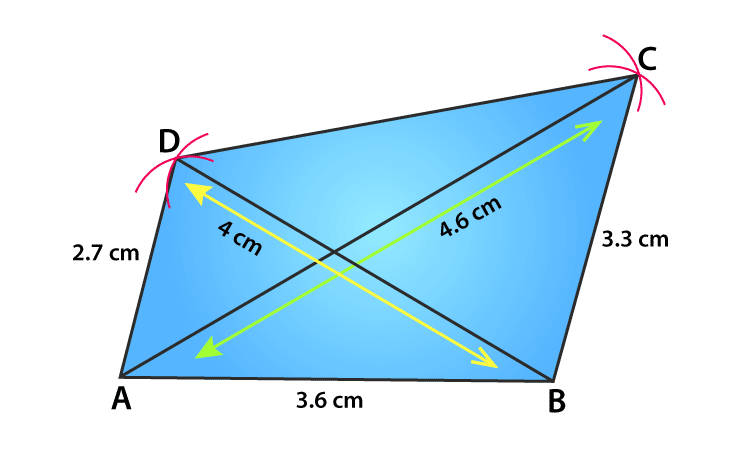5. Construct a quadrilateral PQRS in which QR=7.5cm, PR=PS=6cm, RS=5cm, and QS=10cm. measure the fourth side.

Solution: The given Quadrilateral PQRS can be drawn by following the steps.

Step1: Draw a line QR= 7.5cm

Step2: Draw an arc of 10cm from Q

Step3: From R with 5cm as radius cut the arc drawn in step2 and that point of intersection is S.

Step4: Draw another arc from R with 6cm of radius

Step5: From S take 6cm as radius and cut the arc drawn in step4 and the point of intersection is P. now join PR and PS.

∴ The constructed quadrilateral looks like: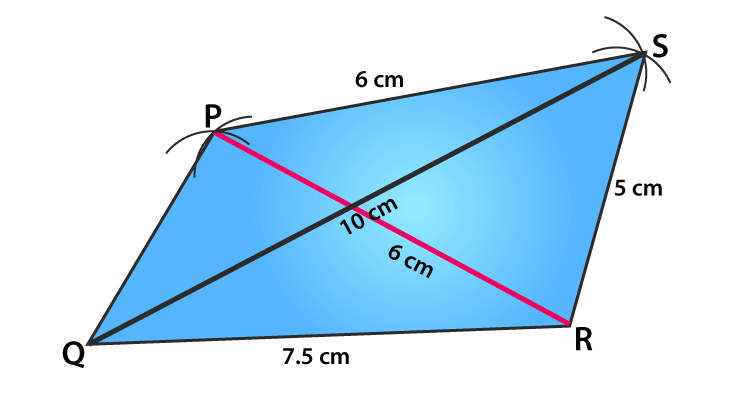6. Construct a quadrilateral ABCD in which AB=3.4cm, CD=3cm, DA=5.7cm, AC=8cm and BD= 4cm.

Solution: The given Quadrilateral ABCD can be drawn by following the steps.

Step1: Draw a line BD= 4cm

Step2: Draw an arc of 3.4cm from B

Step3: from D take radius of 5.7cm and cut the arc drawn in step2 and that point of intersection is A.

Step4: Draw another arc from D with 3cm as radius.

Step5: From A take 8cm and cut the arc drawn in step4 and the point of intersection is C. now join CD and AC.

∴ The constructed quadrilateral looks like: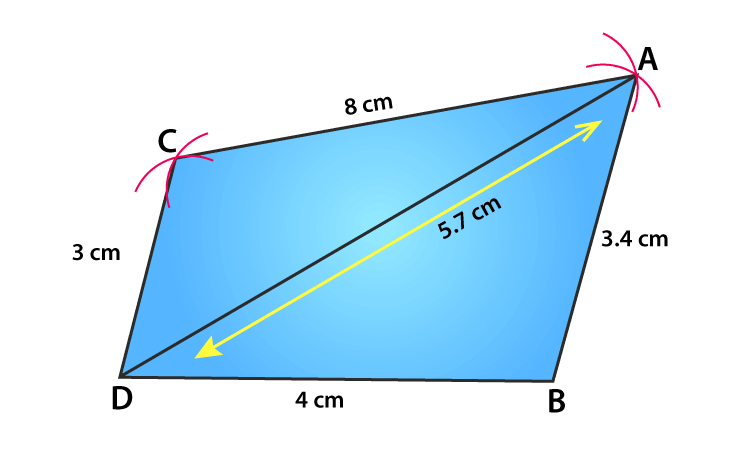### Access other Exercise of RS Aggarwal Solutions for Class 8 Maths Chapter 17- Construction of Quadrilaterals

Exercise 17B Solutions 16 Questions

## RS Aggarwal Solutions for Class 8 Maths Chapter 17- Construction of Quadrilaterals Exercise 17A

Exercise 17A of RS Aggarwal Class 8, Construction of Quadrilaterals. This exercise mainly deals with questions related to constructing different Quadrilaterals.

Students are suggested to practice the problems on a regular basis which will help them excel in their exams and increase their overall percentage. Practicing as many times as possible helps in building time management skills and also boosts the confidence level to achieve high marks.